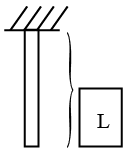# ISRO Scientist or Engineer Refrigeration & Air-Conditioning 2013

Instructions

For the following questions answer them individually

Question 1

# The resultant of two equal forces P making an angle $$\theta$$, is given by:Question 2

# A body weighs 98N on a spring balance on the north pole. The same body is shifted to the equator, the weight of the body at the equator on the same scale is: (Assume g = 9.8 m/sec$$^2$$ and radius of the earth = 6400 km)Question 3

# A uniform heavy rod of weight W, cross-sectional area A and length L and having Young’s Modulus E is hanging vertically from a fixed support. Neglecting lateral contraction, the elongation of the rod is given by:Question 4

# Two bars B1 and B2 of different materials and the same size are subjected to the same tensile force. If the bars have unit elongation in the ratio of 3:7 respectively, then theratio of modulus ofelasticity of the two bars B1 and B2 will be:Question 5

# The pressure at a point 6 m below the free surface of water is:Question 6

# A uniform body of 4 m long, 1 m wide and 1 m deep floats in water.If the depth of immersion is 0.6 m, then the weight of the body is:Question 7

# A jet of water discharging from a 6 cm diameter orifice has a diameter of 40 mm at its vena contracta. The coefficient of contraction is:Question 8

# A Pelton wheel develops 2000 kW under a head of 100 metres while running at 200 r.p.m. and discharging 3000 litres of water per second. The unit discharge of the wheel is:Question 9

# What Drag force is exerted at sea level by a 3.5m braking Parachute when the speed is 20 m/s $$C_d = 1.20$$ and this remains constant, $$\rho$$(sea level) = 1.225 kg/m$$^3$$Question 10

# To convert volumetric analysis to gravimetric analysis, the relative volume of each constituent of the flue gases is:OR